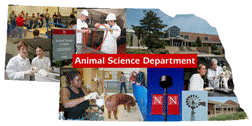## Animal Science Department## Faculty Papers and Publications in Animal Science

#### Date of this Version

June 1991

Published in Journal of Dairy Science Vol. 74, No. 12, 1991.

#### Abstract

Estimation of (co)variance components by derivative-free REML requires repeated evaluation of the log-likelihood function of the data. Gaussian elimination of the augmented mixed model coefficient matrix is often used to evaluate the likelihood function, but it can be costly for animal models with large coefficient matrices. This study investigated the use of a direct sparse matrix solver to obtain the log-likelihood function. The sparse matrix package SPARSPAK was used to reorder the mixed model equations once and then repeatedly to solve the equations by Cholesky factorization to generate the terms required to calculate the likelihood. The animal model used for comparison contained 19 fixed levels, 470 maternal permanent environmental effects, and 1586 direct and 1586 maternal genetic effects, resulting in a coefficient matrix of order 3661 with .3% nonzero elements after including numerator relationships. Compared with estimation via Gaussian elimination of the unordered system, utilization of SPARSPAK required 605 and 240 times less central processing unit time on mainframes and personal computers, respectively. The SPARSPAK package also required less memory and provided solutions for all effects in the model.

COinS